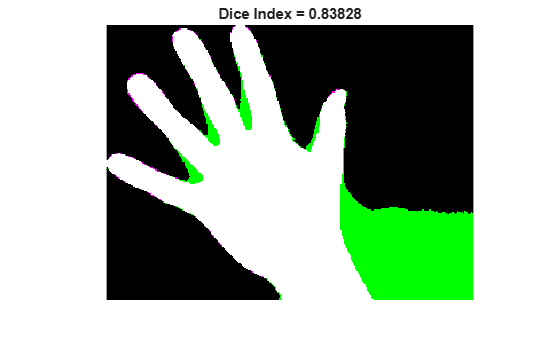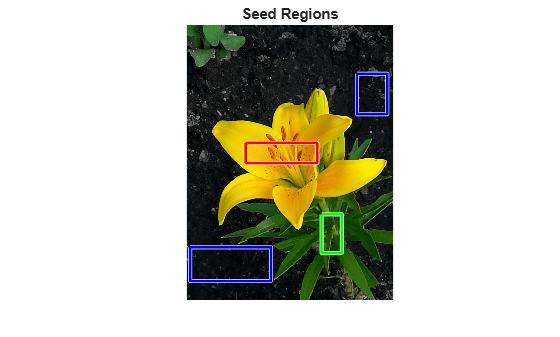# dice

Sørensen-Dice similarity coefficient for image segmentation

## Syntax

``similarity = dice(BW1,BW2)``
``similarity = dice(L1,L2)``
``similarity = dice(C1,C2)``

## Description

example

````similarity = dice(BW1,BW2)` computes the Sørensen-Dice similarity coefficient between binary images `BW1` and `BW2`.```

example

````similarity = dice(L1,L2)` computes the Dice index for each label in label images `L1` and `L2`.```
````similarity = dice(C1,C2)` computes the Dice index for each category in categorical images `C1` and `C2`.```

## Examples

collapse all

Read an image with an object to segment. Convert the image to grayscale, and display the result.

```A = imread('hands1.jpg'); I = im2gray(A); figure imshow(I) title('Original Image')```Use active contours (snakes) to segment the hand.

```mask = false(size(I)); mask(25:end-25,25:end-25) = true; BW = activecontour(I, mask, 300);```

Read in the ground truth segmentation.

`BW_groundTruth = imread('hands1-mask.png');`

Compute the Dice index of the active contours segmentation against the ground truth.

`similarity = dice(BW, BW_groundTruth);`

Display the masks on top of each other. Colors indicate differences in the masks.

```figure imshowpair(BW, BW_groundTruth) title(['Dice Index = ' num2str(similarity)])```This example shows how to segment an image into multiple regions. The example then computes the Dice similarity coefficient for each region.

Read an image with several regions to segment.

`RGB = imread('yellowlily.jpg');`

Create scribbles for three regions that distinguish their typical color characteristics. The first region classifies the yellow flower. The second region classifies the green stem and leaves. The last region classifies the brown dirt in two separate patches of the image. Regions are specified by a 4-element vector, whose elements indicate the x- and y-coordinate of the upper left corner of the ROI, the width of the ROI, and the height of the ROI.

```region1 = [350 700 425 120]; % [x y w h] format BW1 = false(size(RGB,1),size(RGB,2)); BW1(region1(2):region1(2)+region1(4),region1(1):region1(1)+region1(3)) = true; region2 = [800 1124 120 230]; BW2 = false(size(RGB,1),size(RGB,2)); BW2(region2(2):region2(2)+region2(4),region2(1):region2(1)+region2(3)) = true; region3 = [20 1320 480 200; 1010 290 180 240]; BW3 = false(size(RGB,1),size(RGB,2)); BW3(region3(1,2):region3(1,2)+region3(1,4),region3(1,1):region3(1,1)+region3(1,3)) = true; BW3(region3(2,2):region3(2,2)+region3(2,4),region3(2,1):region3(2,1)+region3(2,3)) = true;```

Display the seed regions on top of the image.

```imshow(RGB) hold on visboundaries(BW1,'Color','r'); visboundaries(BW2,'Color','g'); visboundaries(BW3,'Color','b'); title('Seed Regions')```Segment the image into three regions using geodesic distance-based color segmentation.

`L = imseggeodesic(RGB,BW1,BW2,BW3,'AdaptiveChannelWeighting',true);`

Load a ground truth segmentation of the image.

`L_groundTruth = double(imread('yellowlily-segmented.png'));`

Visually compare the segmentation results with the ground truth.

```figure montage({label2rgb(L),label2rgb(L_groundTruth)}) title('Comparison of Segmentation Results (Left) and Ground Truth (Right)')```Compute the Dice similarity index for each segmented region. The Dice similarity index is noticeably smaller for the second region. This result is consistent with the visual comparison of the segmentation results, which erroneously classifies the dirt in the lower right corner of the image as leaves.

`similarity = dice(L, L_groundTruth)`
```similarity = 3×1 0.9396 0.7247 0.9139 ```

## Input Arguments

collapse all

First binary image, specified as a logical array of any dimension.

Data Types: `logical`

Second binary image, specified as a logical array of the same size as `BW1`.

Data Types: `logical`

First label image, specified as an array of nonnegative integers, of any dimension.

Data Types: `double`

Second label image, specified as an array of nonnegative integers, of the same size as `L1`.

Data Types: `double`

First categorical image, specified as a `categorical` array of any dimension.

Data Types: `category`

Second categorical image, specified as a `categorical` array of the same size as `C1`.

Data Types: `category`

## Output Arguments

collapse all

Dice similarity coefficient, returned as a numeric scalar or numeric vector with values in the range [0, 1]. A `similarity` of 1 means that the segmentations in the two images are a perfect match. If the input arrays are:

• binary images, `similarity` is a scalar.

• label images, `similarity` is a vector, where the first coefficient is the Dice index for label 1, the second coefficient is the Dice index for label 2, and so on.

• categorical images, `similarity` is a vector, where the first coefficient is the Dice index for the first category, the second coefficient is the Dice index for the second category, and so on.

Data Types: `double`

collapse all

### Dice Similarity Coefficient

The Dice similarity coefficient of two sets A and B is expressed as:

`dice`(A,B) = 2 * | `intersection`(A,B) | / ( | A | + | B | )

where |A| represents the cardinal of set A. The Dice index can also be expressed in terms of true positives (TP), false positives (FP) and false negatives (FN) as:

`dice`(A,B) = 2 * TP / ( 2 * TP + FP + FN)

The Dice index is related to the Jaccard index according to:

`dice`(A,B) = 2 * `jaccard`(A,B) / (1 + `jaccard`(A,B) )